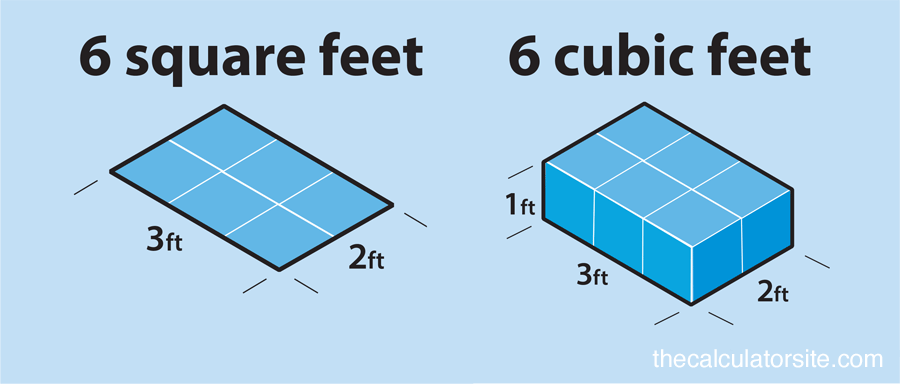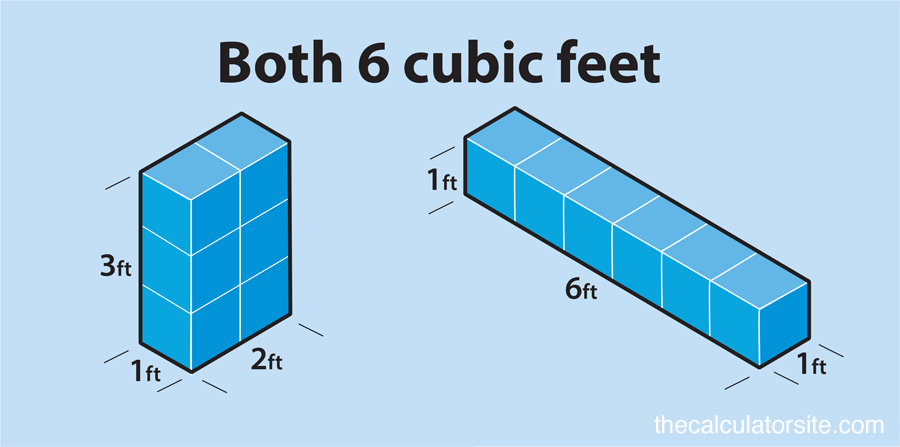Calc

# Square Feet to/from Cubic FeetUse this calculator tool to convert between square feet and cubic feet. Should you wish to calculate cubic feet for a width, length and height, please use the cubic feet calculator. To calculate the square feet of an object using width and length, try the square footage calculator.

## Cubic feet to square feet

#### Disclaimer

Whilst every effort has been made in building this square feet and cubic feet calculator, we are not to be held liable for any special, incidental, indirect or consequential damages or monetary losses of any kind arising out of or in connection with the use of the converter tools and information derived from the web site. This square feet and cubic feet calculator is here purely as a service to you, please use it at your own risk. Do not use calculations for anything where loss of life, money, property, etc could result from inaccurate conversions.

## How do I convert square feet to cubic feet?

Before we discuss how to do this calculation, it is important to note that the square foot is a unit of area (two dimensions) and the cubic foot is a unit of volume (three dimensions). So, it is not a conversion as such, as we are not comparing like for like.It's worth noting that we have a separate calculator for calculating cubic yards from square feet.

To calculate cubic feet based upon a square feet figure requires us to know an extra dimension: height/depth. You could, in theory, fit many different measurements of square feet into a cubic foot. Picture a swimming pool - it could be very long, wide and shallow. Or, it could be shorter in length and width and be very deep. Both of these swimming pools could, in theory, have the same cubic feet volume. But, their surface area would be very different. Here's an example of two boxes, both measuring 6 cubic feet.Your reasons for wanting to calculate cubic feet from a square feet figure may vary. One common reason is for landscaping. You might want to buy mulch or topsoil for your garden - these materials are commonly sold in cubic feet. Let's assume you know how many square feet your garden measures, now you need to work out how many cubic feet of topsoil you'll need. Here's what we need to know:

Note: these formulae assume all measurements are in feet.

### Formula for cubic feet

Cubic feet = width × length × height (or depth)

### Square feet to cubic feet

Cubic feet = square feet × height (or depth)

### Example

Let's say you have a garden measuring 20 square feet, and you want to cover it in 3 inches of topsoil. The topsoil you want is sold by the cubic foot, so we need to do a conversion and calculation.

1. Convert our depth figure from inches to feet (so that units are the same). There are 12 inches in a foot. 3 ÷ 12 = 0.25 ft.
Our topsoil depth is 0.25 ft.
2. Cubic feet = square feet × depth.
So: 20 × 0.25 = 5.
3. We have our total: 5 cubic feet.

## How do I convert cubic feet to square feet?

As we discussed above, it is important to note that this is not a conversion, due to the fact that a cubic foot is a unit of volume (three dimensions) and a square foot is a unit of area (two dimensions). So, you're not comparing like for like. In order to calculate how many square feet of area there are in your cubic feet of volume, you need to know one of the dimensions. In our calculator, above, we've stated this as 'height', but it could equally be length or width, depending on which side of the object you're trying to measure. Once you have one of the dimensions, your calculations can be carried out using the formulae below.

Note: these formulae assume all measurements are in feet.

### Formula for square feet

Square feet = width (ft) × length (ft)

### Cubic feet to square feet

Square feet = cubic feet ÷ height (ft)

### Example

Using our topsoil example above, and working backwards, you'll see how it works. As a reminder, we have 5 cubic feet of top soil and a depth of 0.25 feet (3 inches). What is the square feet measurement?
1. Square feet = cubic feet ÷ depth.
So: 5 ÷ 0.25 = 20.
2. We have our total: 20 square feet.

Of course, if you use our calculator at the top of the page, it'll work these calculations out for you.